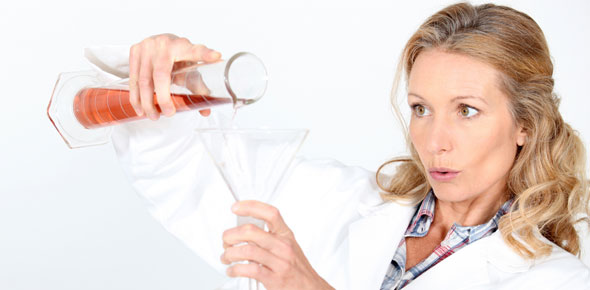# Unit 7 - Chemical Reactions Review

24 Questions | Attempts: 143SettingsIn science, a chemical reaction is a process that leads to the transformation of one set of chemical substances to another. This typically involves the changing of electron positions in the manipulation of chemical bonds between atoms.

• 1.
What type of reaction produces a precipitate?
• A.

Synthesis

• B.

Single displacement

• C.

Double displacement

• D.

Decomposition

• 2.
____ is a way to describe a chemical reaction using chemical formulas and symbols.
• A.

Synthesis

• B.

A physical law

• C.

The law of conservation of matter

• D.

A chemical equation

• 3.
An insoluble compound that comes out of solution during a double displacement reaction is called a _____.
• A.

Reactant

• B.

Solvent

• C.

Precipitate

• D.

Catalyst

• 4.
What does it mean for a chemical equation to be balanced?
• A.

The number of reactants is equal to the number of products.

• B.

The reactants and products are written with the same coefficients.

• C.

The same number of atoms of each element is on both sides of the equation.

• D.

The solid products weight the same as the reactants.

• 5.
In chemical equations, ______ represents the number of units of each substance.
• A.

Precipitates

• B.

Products

• C.

Reactants

• D.

Coefficients

• 6.
The chemical formula for a compound tells _____.
• A.

How stable the compound is

• B.

What type of chemical bond it forms

• C.

How electrons are arranged

• D.

What elements it contains

• 7.
If electric current passes through water and initiates a decomposition reaction, what are the expected products?
• A.

Oxygen and water

• B.

Hydrogen and oxygen

• C.

Hydrogen peroxide

• D.

Hydrogen oxide

• 8.
When iron combines with oxygen and water in the air to form rust, what substance in the reaction is the product?
• A.

Iron

• B.

Rust

• C.

Water

• D.

Oxygen

• 9.
As a result of the experiments of Lavoisier, you know that in a chemical reaction the masses of the products _____.
• A.

Is less than the mass of the reactants

• B.

Always equals the mass of the reactants

• C.

Cannot be predicted based on the mass of the reactants

• D.

Is twice as great as the mass of the reactants

• 10.
New substances produced by a chemical reaction are ____.
• A.

Products

• B.

Resultants

• C.

Reactants

• D.

Coefficients

• 11.
In a ______ reaction, two or more substances combine to form another substance.
• A.

Single displacement

• B.

Double displacement

• C.

Decomposition

• D.

Synthesis

• 12.
A change in which one or more substances are converted into new substances is a _____.
• A.

Nuclear reaction

• B.

Chemical reaction

• C.

Chemical fusion

• D.

Physical change

• 13.
A(n) _____ is a substance that speeds up a chemical reaction without being permanently changed itself.
• A.

Catalyst

• B.

Inhibitor

• C.

Exotherm

• D.

Endotherm

• 14.
Why is oxygen written with a subscript 2?
• A.

It usually exists as a diatomic molecule.

• B.

Its mass is twice as great as the reactants'.

• C.

There are two times as many oxygen atoms as reactant atoms.

• D.

Its mass increases by two times as a result of the reaction.

• 15.
The law of conservation of mass states that _____.
• A.

In a chemical reaction, matter is not created or destroyed, but is conserved.

• B.

Matter can be created and destroyed but does not change forms.

• C.

In a chemical reaction, efforts should be made to preserve rare elements without changing them.

• D.

In a chemical reaction, the final mass of the products is always greater than the starting mass of the reactants.

• 16.
Which of the following is a chemical reaction?
• A.

Water freezing into ice

• B.

Water evaporating from salt water leaving salt crystals

• C.

Dew forming on grass

• D.

Rust forming on a car body

• 17.
Some elements usually exist in combinations of two atoms. These combinations are called ______.
• A.

Diatomic molecules

• B.

Coefficients

• C.

Ions

• D.

Isotopes

• 18.
Identify the following as either endothermic or exothermic. The temperature of the beaker holding the reacting substances is increased.
• A.

Endothermic

• B.

Exothermic

• 19.
Identify the following as either endothermic or exothermic. The temperature of the beaker holding the reacting substances is decreased.
• A.

Endothermic

• B.

Exothermic

• 20.
Identify the following as either endothermic or exothermic. Frost formed on the outside of the beaker holding the reacting substances.
• A.

Endothermic

• B.

Exothermic

## Related TopicsBack to top
×

Wait!
Here's an interesting quiz for you.